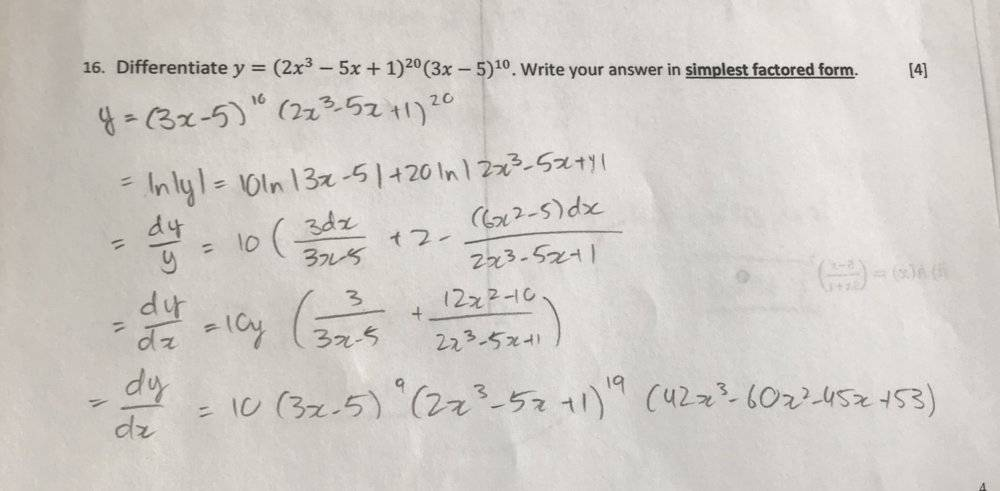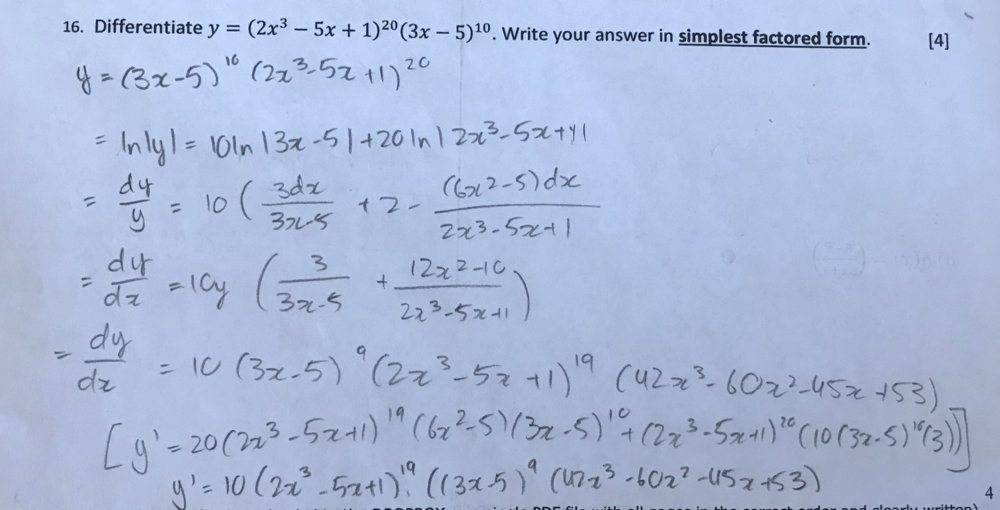# Differentiate 𝑦 = (2𝑥^3 − 5𝑥 + 1)^20(3𝑥 − 5)^10

ttpp1124
Homework Statement:
Differentiate 𝑦 = (2𝑥^3 − 5𝑥 + 1)^20(3𝑥 − 5)^10. Write your answer in simplest factored form.
I've solved it..but I feel like it can be factored further?
Relevant Equations:
n/aLast edited by a moderator:

Homework Helper
I don't see any reason to use "logarithmic integration". Just the "product rule" and "chain rule" are sufficient.
$$y= (2𝑥^3 − 5𝑥 + 1)^{20}(3𝑥 − 5)^{10}$$

$$y'= 20(2x^3- 5x+ 1)^{19}(6x^2- 5)(3x- 5)^{10}+ (2x^3- 5x+ 1)^{20}(10(3x- 5)^{10}(3))$$.

Both third degree polynomials you end up with have real roots (they are polynomials of odd degree). I don't expect that you can completely factorise these into linear/quadratic factors as the roots will probably be quite ugly.

ttpp1124
I don't see any reason to use "logarithmic integration". Just the "product rule" and "chain rule" are sufficient.
$$y= (2𝑥^3 − 5𝑥 + 1)^{20}(3𝑥 − 5)^{10}$$

$$y'= 20(2x^3- 5x+ 1)^{19}(6x^2- 5)(3x- 5)^{10}+ (2x^3- 5x+ 1)^{20}(10(3x- 5)^{10}(3))$$.
wow, this question can really be solved in one step like that?

ttpp1124
I guess I will leave it as it is then.
Both third degree polynomials you end up with have real roots (they are polynomials of odd degree). I don't expect that you can completely factorise these into linear/quadratic factors as the roots will probably be quite ugly.

Homework Helper
wow, this question can really be solved in one step like that?
Well, the quicker you try to do it, the more likely you are to make mistakes so perhaps you should check my calculations!

•SammyS
Homework Helper
Gold Member
But it isn't in best factored form yet.

Homework Helper

Homework Helper
Gold Member
Look at the statement of the problem in post #1.

ttpp1124sorry for the late reply, but I think I got it..?

Athenian
Just differentiating the equation via a calculator I got:
$$y' = 20\left(2x^3-5x+1\right)^{19}\left(6x^2-5\right)\left(3x-5\right)^{10}+30\left(3x-5\right)^9\left(2x^3-5x+1\right)^{20}$$

or

$$y' = 10(3x-5)^9 (2x^3 - 5x +1)^{19} (42x^3 - 60x^2 - 45x + 53)$$

If you ever just want to double-check your answers, you could always use either symbolab.com or wolframalpha.com. If your university provides students with the pro (i.e. paid) version of WoframAlpha, you could even check your work. Needless to say, it's highly recommended to do your work by hand first before checking the step-by-step solution process which WoframAlpha Pro provides. Note that Symbolab also has the step-by-step solution process too. However, to my knowledge, WolframAlpha is much more versatile and powerful over all. If anything, though, these online calculators are a great tool to double-check your answers.

Homework Helper
Gold Member
If you just write the problem in the first place as differentiate y = u20v10 it is more obvious and easy.